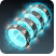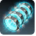# [exact_damage_test]Conditions

## Related effects, buffs and debuffs

• [0s] [not displayed]Effect #1

 Slot: Debuff Duration: 0s Tick rate: does not tick # occurrences: 0
• On Apply

Perform the following actions:

• Play appearance epp . bounty_hunter . energy_burst . instant_cast_2charges, dependent on calling effect
• Spell Damage
- Unknown (609) = (bool) true
- Damage Type = (int) 3
- Slot = (int) 1
- Spell Type = (int) 2
- Level Cap = (int) 0
- Amount Max = (float) 10000
- Amount Min = (float) 10000
- Amount Percent = (float) 0
- Threat Percent = (float) 0
- Standard Health Percent Max = (float) 0
- Standard Health Percent Min = (float) 0
- Amount Modifier Percent = (float) 0
- Coefficient = (float) 0
- Health Steal Percentage = (float) 0
• On Apply

Only when the following conditions are met:

• If previous subeffect did not meet all conditions

Perform the following actions:

• Weapon Damage
- Is Special Ability = (bool) true
- Ignore Dual Wield Modifier = (bool) true
- Unknown (609) = (bool) false
- Flurry Blows Min = (int) 1
- Flurry Blows Max = (int) 1
- Level Cap = (int) 0
- Threat Percent = (float) 0
- Standard Health Percent Max = (float) 0
- Standard Health Percent Min = (float) 0
- Amount Modifier Fixed Max = (float) 10000
- Amount Modifier Fixed Min = (float) 10000
- Amount Modifier Percent = (float) -1
- Coefficient = (float) 0
- Health Steal Percentage = (float) 0
- Slots = Int(5)
• Weapon Damage
- Is Special Ability = (bool) true
- Ignore Dual Wield Modifier = (bool) true
- Unknown (609) = (bool) false
- Flurry Blows Min = (int) 1
- Flurry Blows Max = (int) 1
- Level Cap = (int) 0
- Threat Percent = (float) 0
- Standard Health Percent Max = (float) 0
- Standard Health Percent Min = (float) 0
- Amount Modifier Fixed Max = (float) 1000
- Amount Modifier Fixed Min = (float) 1000
- Amount Modifier Percent = (float) -1
- Coefficient = (float) 0
- Health Steal Percentage = (float) 0
- Slots = Int(2)